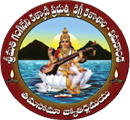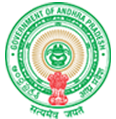# S G K GOVERNMENT DEGREE COLLEGE

#### NAAC with 'B'#### Maths Club

MATHS CLUB

(think before you ink)

AIMS :

• Active involvement of students

• Introduction of new patterns of learning

• Changing the apprehensive attitude

OBJECTIVES :

• Introduction of new patterns of learning

• Awaring students the importance of mathematics in the daily life

• Inspiring the students by reminding the efforts of Mathematicians

• Dispelling unwanted fears from their minds in learning Mathematics

• Orient the students towards practice centric tasks

ACTIVITIES:

1. Solving problems by all possible methods

2. Observing all the prominent days of Mathematics

3. Conduction of student seminars, quizzes, debates, solving

Tricky problems

4. Asking students to prepare problems under assignments

5. Revise basics of mathematics